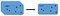# An Introduction to Dimensionality Reduction for Visualization Using t-SNE

In this article I will be giving an introduction of t-SNE (t-distributed Stochastic Neighbor Embedding), which is a recent state-of-art dimensionality reduction technique for achieving a 2-dimensional easily visualizable and presentable view of data without hampering the relationship between data points. I will NOT be going into deep mathematical logic of t-SNE, but, instead would be giving just an overview using an example. However, at a later point of time, I will be explaining the implementation of t-SNE in Python using a high dimensional dataset as an example in a follow up article.Dimensionality Reduction by preserving global structure of data (Example: PCA) — Image source: Google## Shobhit Gupta

Aspiring Data Scientist and Machine Learning Engineer